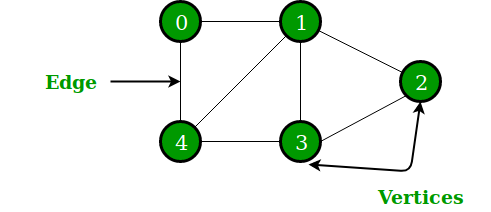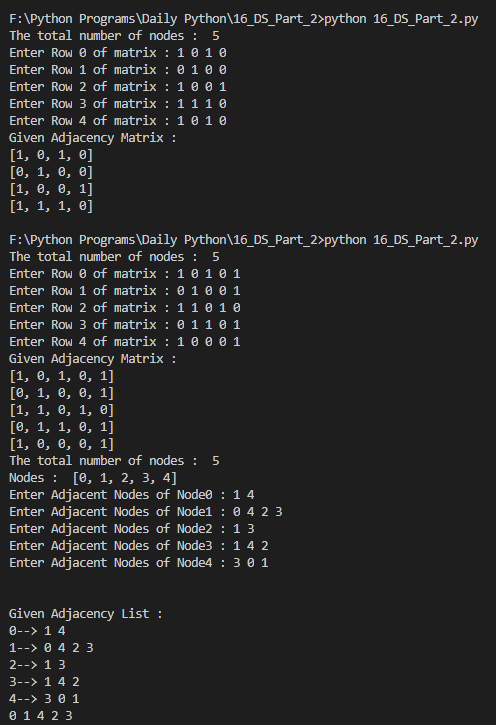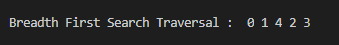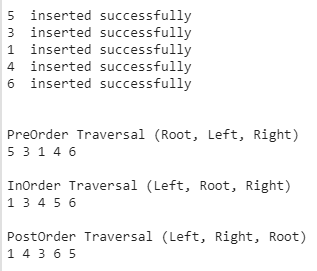# Implementing basic Data Structures in Python — Part 2| Daily Python #16

This article is a part of Daily Python challenge that I have taken up for myself. I will be writing short python articles daily.

# Graphs

A Graph is a non-linear data structure consisting of nodes and edges. The nodes are sometimes also referred to as vertices and the edges are lines or arcs that connect any two nodes in the graph. Formally a Graph can be defined as — A Graph consists of a finite set of vertices(or nodes) and set of Edges that connect a pair of nodes.In the above Graph, the set of vertices V = {0,1,2,3,4} and the set of edges E = {01, 12, 23, 34, 04, 14, 13}.

## Two most commonly used representations of Graphs:

We will cover both of these representations in this article.

It is a 2D array of size V x V where V is the number of vertices in a graph.
Let the 2D array be matrix[][], a slot matrix[i][j] = 1 indicates that there is an edge from vertex i to vertex j.

The adjacency matrix for an undirected graph is always symmetric.
Adjacency Matrix is also used to represent weighted graphs. If matrix[i][j] = w, then there is an edge from vertex i to vertex j with weight w.

An array of lists is used. The size of the array is equal to the number of vertices. Let the array be an array[]. An entry array[i] represents the list of vertices adjacent to the ith vertex. This representation can also be used to represent a weighted graph. The weights of edges can be represented as lists of pairs.

Following is the adjacency list representation of the above graph.

## Let’s define a class Graph that will implement both of the above representations

`class Graph:    def __init__(self,totalNodes):        self.totalNodes = totalNodes        self.adjMatrix = []        self.adjList = []    def acceptAdjMatrix(self):        print('The total number of nodes : ',self.totalNodes)        for row in range(self.totalNodes):            temp = list(map(int,input('Enter Row ' +str(row)+' of matrix : ').split(' ')))            self.adjMatrix.append(temp)            def printAdjMatrix(self):        if len(self.adjMatrix) == 0:            print('Please fill the Adjacency Matrix')            return         print('Given Adjacency Matrix :')        for row in self.adjMatrix:            print(row)    def acceptAdjList(self):        print('The total number of nodes : ',self.totalNodes)        print('Nodes : ',list(range(self.totalNodes)))        for node in range(self.totalNodes):            temp = list(map(int,input('Enter Adjacent Nodes of Node' +str(node)+' : ').split(' ')))            self.adjList.append(temp)     def printAdjList(self):        if len(self.adjList) == 0:            print('Please fill the Adjacency List')            return        print('\n\nGiven Adjacency List :')        for i in range(self.totalNodes):            print(i,end='--> ')            for j in self.adjList[i]:                print(j,end=' ')                    print('')`

The above code snippet has the basic functions of accepting the Adjacency Matrix and the Adjacency List, and also printing these two representations.

`graph = Graph(5)graph.acceptAdjMatrix()graph.printAdjMatrix()graph.acceptAdjList()graph.printAdjList()`Snip of the Output of the above code snippet

There are multiple ways to traverse the graphs, one such method is Breadth-First Search Traversal

`    def BFS(self):        iterator = 0        queue = [iterator]        visited = *self.totalNodes        visited[iterator] = 1        while len(queue)>0:            queue.extend([ node for node in self.adjList[iterator] if visited[node] != 1 and node not in queue])            print(queue,end=' ')            visited[iterator] = 1            iterator = queue                       queue = queue[1:]        print('')`Snip of the Output of the above code

# Trees

A tree is a widely used abstract data type (ADT) that simulates a hierarchical tree structure, with a root value and sub-trees of children with a parent node, represented as a set of linked nodes.

A tree data structure can be defined recursively as a collection of nodes (starting at a root node), where each node is a data structure consisting of a value, together with a list of references to nodes (the “children”), with the constraints that no reference is duplicated, and none points to the root.

The most common representation of a tree is the Binary Search Tree

Binary Search Tree is a node-based binary tree data structure which has the following properties:

• The left subtree of a node contains only nodes with keys lesser than the node’s key.
• The right subtree of a node contains only nodes with keys greater than the node’s key.
• The left and right subtree each must also be a binary search tree.

## Let’s define a class for a simple Node that will be the basic element of the tree

`class Node:    def __init__(self,value):    self.value = value    self.rightNode = None    self.leftNode = None`

## Let’s define a class for the Binary Search Tree

`class BSTree:  def __init__(self):    self.headNode = None  def insertNode(self,value):    node = Node(value)    if self.headNode == None:      self.headNode = node      print(value,' inserted successfully')  else:      iterator = self.headNode      while True:        if value < iterator.value:          if iterator.leftNode == None:            iterator.leftNode = node            break          else:            iterator = iterator.leftNode        else:          if iterator.rightNode == None:            iterator.rightNode = node            break          else:            iterator = iterator.rightNode      print(value,' inserted successfully')  def printTree(self):    print('\n\nPreOrder Traversal (Root, Left, Right)')    self.preOrder(self.headNode)      print('\n\nInOrder Traversal (Left, Root, Right)')    self.inOrder(self.headNode)      print('\n\nPostOrder Traversal (Left, Right, Root)')    self.postOrder(self.headNode)    def inOrder(self,node):    if node == None:      return    self.inOrder(node.leftNode)    print(node.value, end=' ')    self.inOrder(node.rightNode)  def preOrder(self,node):    if node == None:      return    print(node.value, end=' ')    self.preOrder(node.leftNode)    self.preOrder(node.rightNode)  def postOrder(self,node):    if node == None:      return    self.postOrder(node.leftNode)    self.postOrder(node.rightNode)    print(node.value, end=' ')`

## Depth First Traversals:

1. Inorder (Left, Root, Right)
2. Preorder (Root, Left, Right)
3. Postorder (Left, Right, Root)

## Let’s test the above code by inserting few values in the tree and traverse it

`tree = BSTree()tree.insertNode(5)tree.insertNode(3)tree.insertNode(1)tree.insertNode(4)tree.insertNode(6)tree.printTree()`Snip of the Output of the above code snippet

I hope this article was helpful, do leave some claps if you liked it.

--

--